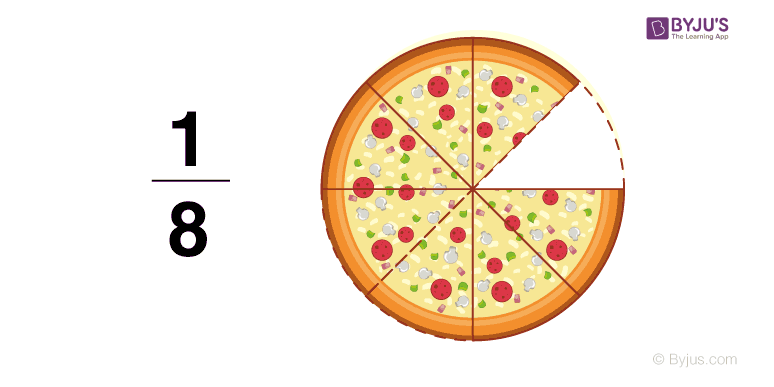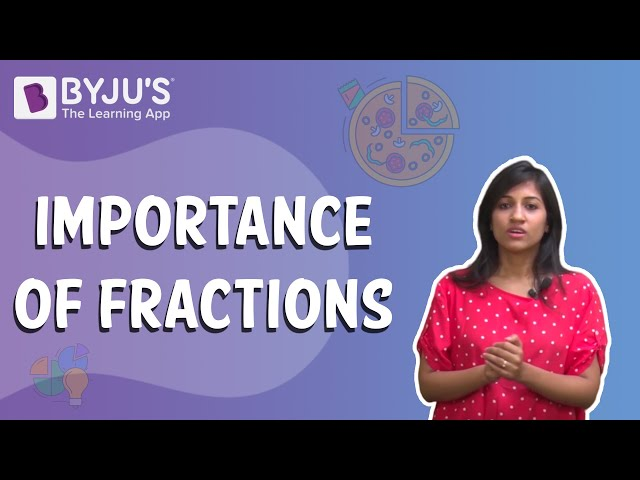# Fractions Worksheets

Fractions Worksheets are designed to improve the mathematical skills of the students who want to learn more about Fractions. These worksheets cover all the basic concepts of Fractions for the CBSE class 6, 7 and 8 students. Every year, the CBSE or the central board of secondary education conducts the final term, summative assessments 1 and 2 for all the CBSE schools. These maths worksheets for class 7 are compiled to help students score good marks in the exam.

All the questions in the worksheets are based on the NCERT and the CBSE Syllabus Guidelines. The students need to brush up with the basic concepts before referring to these worksheets for class 8 to gain excellence to score more marks in the CBSE examination.

Let’s have a brief introduction to fractions.

A fraction represents a numerical value, which defines the parts of a whole. It can be written in its simplified form if the numerator and denominator have no common factor. In other words, it is impossible to find a number that is a divisor to both the numerator and the denominator in a fraction’s simplified form. A fraction is said to be in the simplest (or lowest) form if its numerator and the denominator have no common factor except 1.

A common fraction is technically the correct term. We will use a fraction in these materials if there is no scope for confusion. The word fraction comes from the Latin stem fraction, which means “breaking into pieces.”The concept of fractions and the relations between fractions and other types of numbers, like many abstract math formulas and concepts, is not always easy to understand. The techniques for solving both simple and complex equations involving fractions are explained first before solving the problems. Complete worksheets are provided, starting with elementary and basic equations and progressing to complicated equations requiring applying a full range of operations with fractions.

Different types of questions such as single-step questions (requiring a basic knowledge and understanding of the topic presented in the worksheets) and multi-step questions (requiring complete knowledge of all of the concepts presented in the worksheets to that point) are also added.

## Fractions Worksheet For Class 6

 Find ⅝ of 32. Represent each of the given fraction on the number line: a) 3/8 b) 4/7 c) 2/5 Convert the following into mixed fractions: a) 103/12 b) 117/20 c) 81/11 Class 6 Fractions worksheet PDF to solve more questions:- Download PDF Here

## Fractions Worksheet For Class 7

 Find the sum: a) ⅝ + 3/10 b) 37/41 +19/30 c) 2(⅗) +4(7/10) + 2(4/15) What should be added to 5(3/7) to get 12? Find a rectangular park area, which is 41(⅔) m long and 18(⅗) m broad. . Class 7 Fractions worksheet PDF to solve more questions:-Download PDF Here

## Fractions Worksheet For Class 8

 Fill in the blanks with the correct symbol: a) -3/7 ___ 6/-13 b) 0 _____ -3/-5 Subtract ¾ from ⅓, -7 from -4/7. What number should be subtracted from –⅔ to get -⅙? Class 8 Fractions worksheet PDF to solve more questions:- Download PDF Here

Class 6 worksheet on Fractions covers the following concepts.

• A Fraction
• Fraction on the Number Line
• Proper Fractions
• Improper and Mixed Fractions
• Equivalent Fractions
• Simplest Form of a Fraction
• Like Fractions
• Comparing Fractions
• Addition and Subtraction of Fractions

Class 7 worksheet on Fractions covers the following concepts.

• Multiplication of Fractions
• Division of Fractions

## Video Lesson on FractionsTo get more Maths worksheets and practice questions, visit byjus.com and take practice tests for a better understanding of fractions.